# 【学习笔记】机器学习导论5 正则表达式与re库的简单使用

1通用的字符串表达框架
2简洁表达一组字符串的表达式
3判断某字符串的特征归属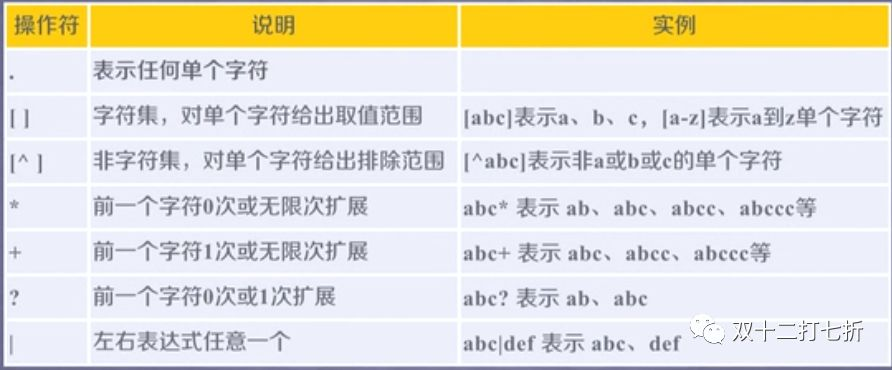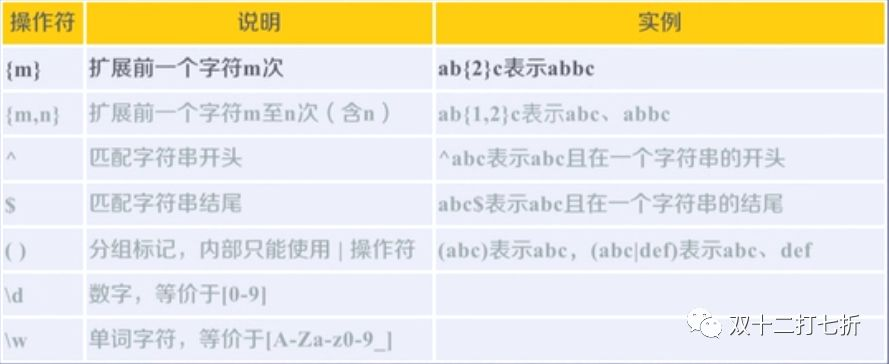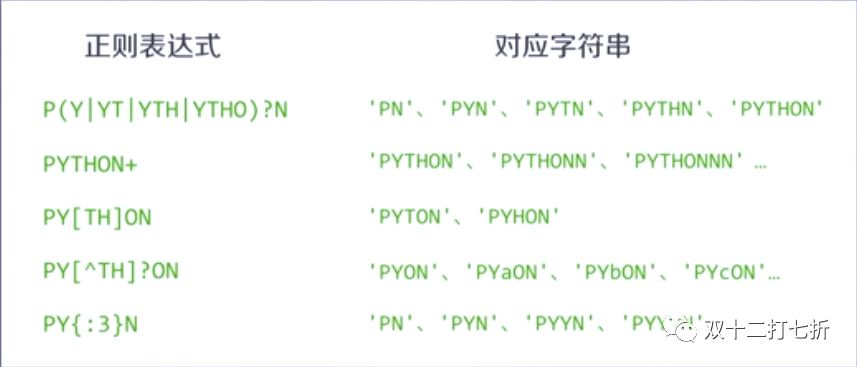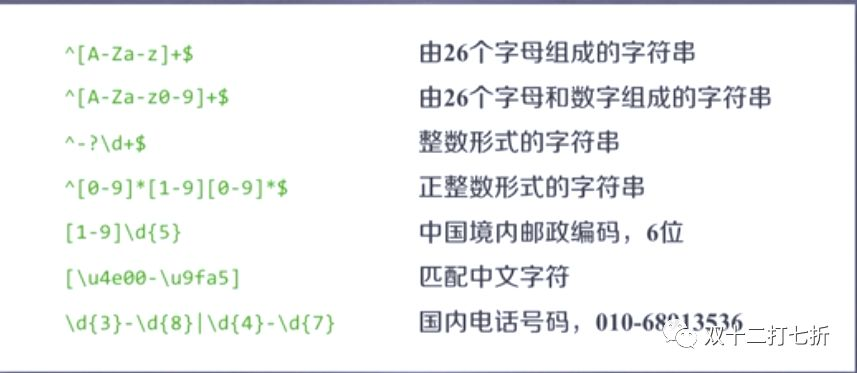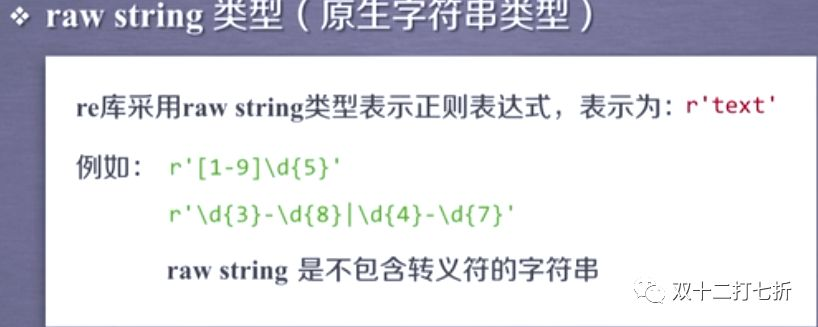re库的主要功能函数：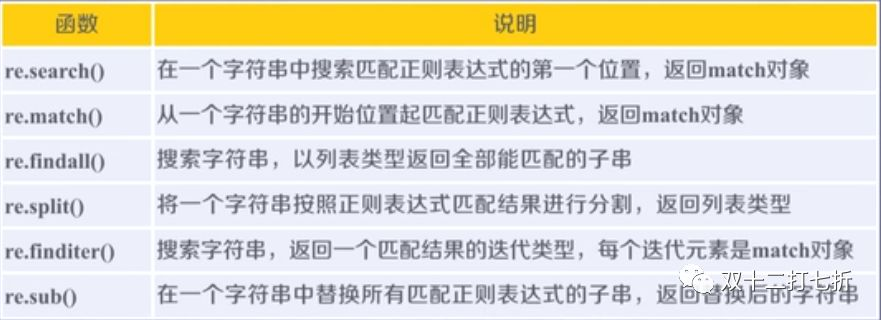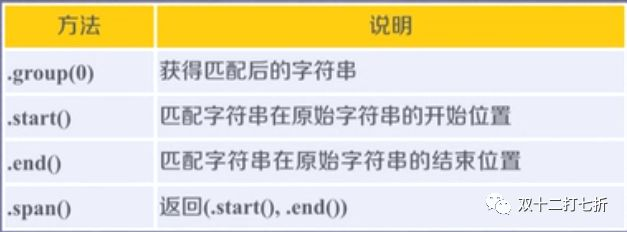re.search(pattern, string, flags=0)

pattern: 正则表达式的字符串或原生字符串表示
string: 待匹配字符串
flag: 正则表达式使用的控制标记

import re
match = re.search(r'[1-9]\d{5}', 'ABCD  999999')  #匹配字符串中的6位数
if match:
print(match.group(0))

999999

re.match(pattern, string, flags=0)

match = re.match(r'[1-9]\d{2}', '999999  ABCD')   #从字符串开始位置匹配第一个3位数
if match:
print(match.group(0))

999

re.findall(pattern, string, flags=0)

ls = re.findall(r'[1-9]\d{5}', 'ABCD1234567  EFGH12345678')   #搜索字符串中所有的六位数
ls

['123456', '123456']

re.split(pattern, string, maxsplit=0, flags=0)

maxsplit: 最大分割数，剩余部分作为最后一个元素输出

ls1 = re.split(r'[1-9]\d{2}', 'ABCD123EFGH1234567')  #以三位数为标志分割字符串
print(ls1)
ls2 = re.split(r'[1-9]\d{5}', 'ABCD123456  EFGH654321', maxsplit=1)  #以六位数为标志分割字符串，只一次
print(ls2)

['ABCD', 'EFGH', '', '7']
['ABCD', '  EFGH654321']

re.finditer(pattern, string, flags=0)

for m in re.finditer(r'[1-9]\d{2}', 'ABC12334567 EFG123456'):   #搜索字符串中所有的三位数，迭代输出
if m:
print(m.group(0))

123
345
123
456

re.sub(pattern, repl, string, count=0, flags=0)

repl: 替换匹配字符串的字符串
count: 匹配的最大的次数

re.sub( r'[1-9]\d{2}', 'EFG', 'ABC123456   abc1234')  #将字符串中所有的三位数用EFG代替

'ABCEFGEFG   abcEFG4'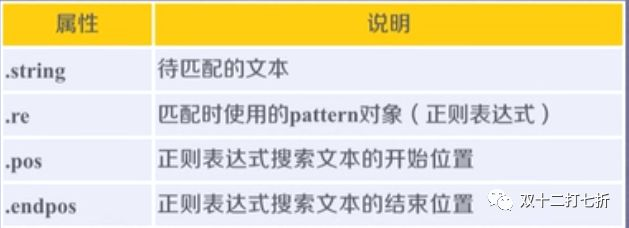import re
match = re.search(r'[1-9]\d{3}', 'ABCD  999999')  #搜索字符串中的第一个4位数
if match:
print(match.group(0))
print(m.string)
print(m.re)
print(m.pos)
print(m.endpos)
print(m.group())

9999
ABC12334567 EFG123456
re.compile('[1-9]\\d{2}')
0
21
456

match.group()

'PYANBNCNDN'

match.group()

'PYAN'